If you have a related question, please click the "Ask a related question" button in the top right corner. The newly created question will be automatically linked to this question.

# OPA189: STABILITY OF BANDPASS FILTER USING OPA189

Part Number: OPA189

Hello,

I have a question about following circuit which i have attached an image of the schematic and the bode plots of CLTF and Loop gain of the circuit. It would be really helpful to get suggestions on the stability of the circuit.

Thank you,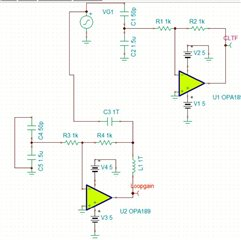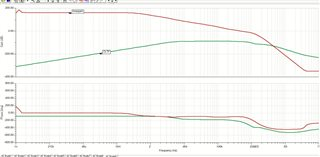• Hi Imanka,

The circuit above is stable with unity gain BW measured at 4.16MHz at 0dB and 81 degree of phase margin. The Vin = (C4/C3)*VG2 approx.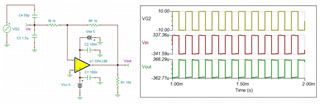OPA189 E2E 03112021.TSC

Best,

Raymond

• Thank you very much raymond. I have a question about shifting the first pole in the CLTF to a much smaller frequency by increasing the values of resistors. Is it possible to do so?

Thank you,

Imanka

• Hi Imanka,

I increase the feedback resistors to 50k and compensated with 3pf capacitor. In the captured schematic below, the unity gain BW is 2.73MHz with phase margin 78 degree, and it is stable.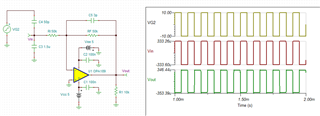How low do you want to reduce the unity gain BW in OPA189? If is in 10-100kHz range, you may consider to use different op amp for G=-1 application.

Best,

Raymond

• Thank you very much Raymond. I actually need the passband gain to be depending on input capacitors C3 and C4 as much as possible. while doing that I also need to minimize the lower cutoff point as low as possible. for an example if the input voltage is a 50Hz signal, i would like to keep the phase shift of the output(with reference to the inevitable phase shift of 180) as small as possible. My understanding was that i should move the lower cutoff point to be small as possible. A bandwidth of 0-20kHz would be sufficient.

Thank you,

Imanka

• Hi Imanka,

I think that this is what you are asking, The bandpass of the circuit is 10-20kHz. I created the pole at approx. 200kHz (1/(2*pi*200kHz*50k) = 15.9 pf, 200kHz is a decade after 20kHz).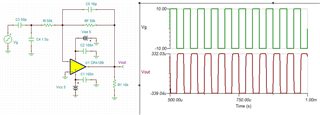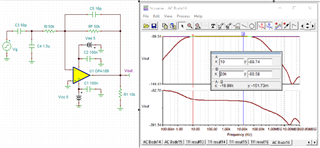OPA189 E2E 03122021B.TSC

Best,

Raymond

• Hi Imanka,

because of your RC input topology a bandwidth of 0Hz...20kHz is impossible: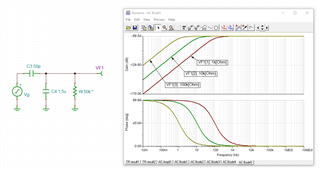imanka_opa189.TSC

Also, why did you choose an inverting configuration? This doesn't go well together with a chopping OPAmp.

And what is your input signal amplitude?

Can you explain your application in more detail?

Kai

• Thank you very much Kai, I intend to use this circuit to accurately measure voltage signals. the capacitive divider at input is to be the part which will determine the attenuation ratio from the input to the output of the OP amp. I didn't try the non-inverting configuration thinking about its voltage gain being always greater than 1.

Thank you,

Imanka